##全国校区# 南方人过冬有多难？用Python带你分析全网取暖器销量数据2020-12-08### CDA数据分析师 出品“你在北方的暖气里四季如春，我在南方的寒冬下冻成冰棍儿”

“北方人过冬靠的是暖气，南方人过冬靠的是一身正气”

“我是一只来自北方的狼，来到南方却被冻成了狗”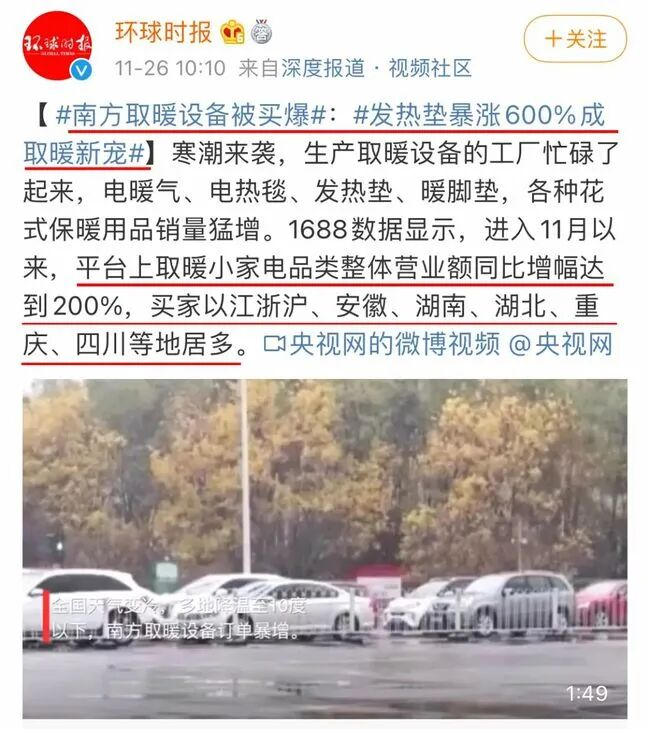1.读取数据

`# 导入工具包 import numpy as np  import pandas as pd  from pyecharts.charts import Bar, Pie, Map, Page from pyecharts import options as opts import jieba `
```# 读取数据 df_all = pd.read_csv('../data/导出数据.csv')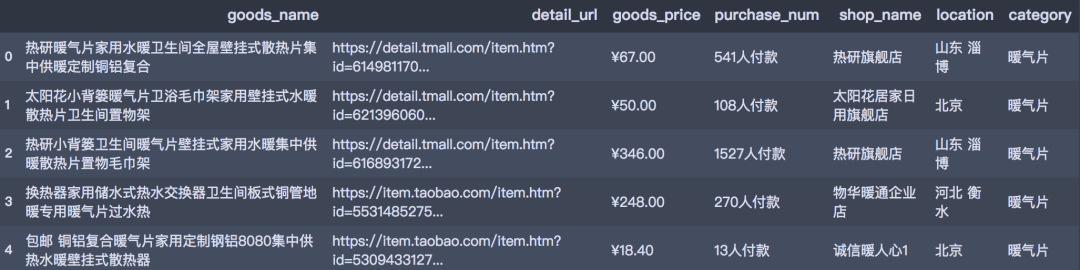`df_all.shape (13212, 7)`

2.数据清洗和整理

• 删除记录的重复值
• goods_price列处理：提取数值
• purchase_num列处理：提取数值
• 计算销售额sales_volume = goods_price*purchase_num
• 删除多余的列

```df = df_all.copy() # 去除重复值 df.drop_duplicates(inplace=True)
df.shape
(6849, 7)```
```# 筛选记录 df = df[df['purchase_num'].str.contains('人付款')] # goods_price列处理 df['goods_price'] = df['goods_price'].str.extract('(d+.{0,1}d*)')
df['goods_price'] = df['goods_price'].astype('float') # purchase_num列处理 df['num'] = df['purchase_num'].str.extract('(d+.{0,1}d*)')
df['num'] = df['num'].astype('float')
df['unit'] = [10000 if '万' in i else 1 for i in df['purchase_num']] # 计算销量 df['purchase_num'] = df['num'] * df['unit'] # 计算销售额 df['sales_volume'] = df['goods_price'] * df['purchase_num'] # 提取省份字段  df['province_name'] = df['location'].astype('str').str.split(' ').apply(lambda x:x)  # 删除多余的列 df.drop(['num', 'unit', 'detail_url'], axis=1, inplace=True) # 重置索引 df = df.reset_index(drop=True)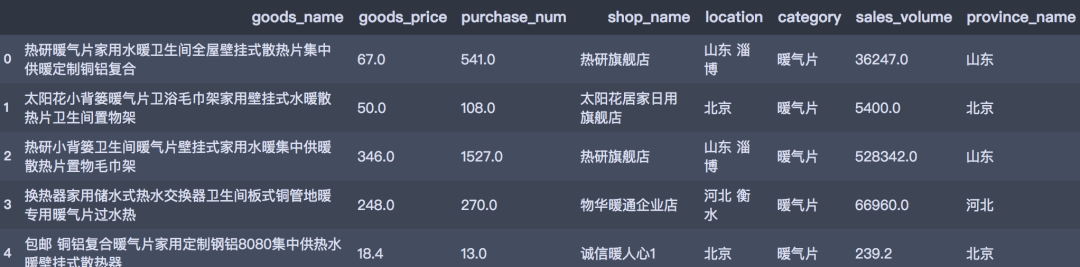3.数据可视化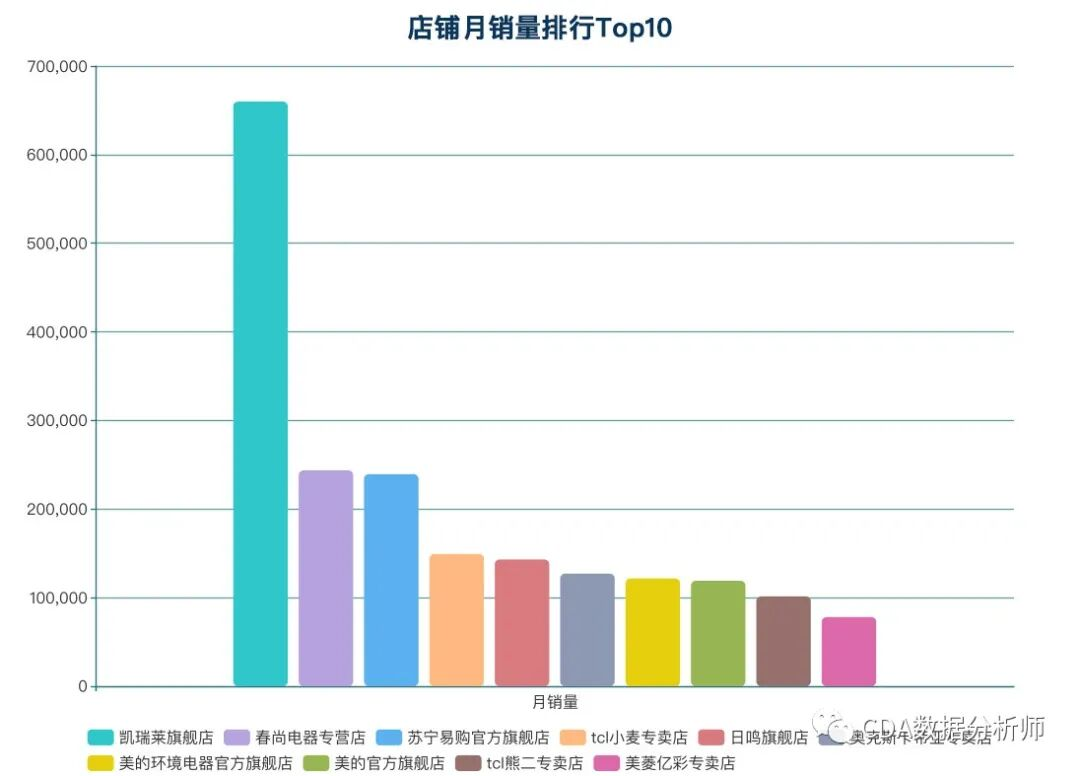`# 计算top10店铺 shop_top10 = df.groupby('shop_name')['purchase_num'].sum().sort_values(ascending=False).head(10)`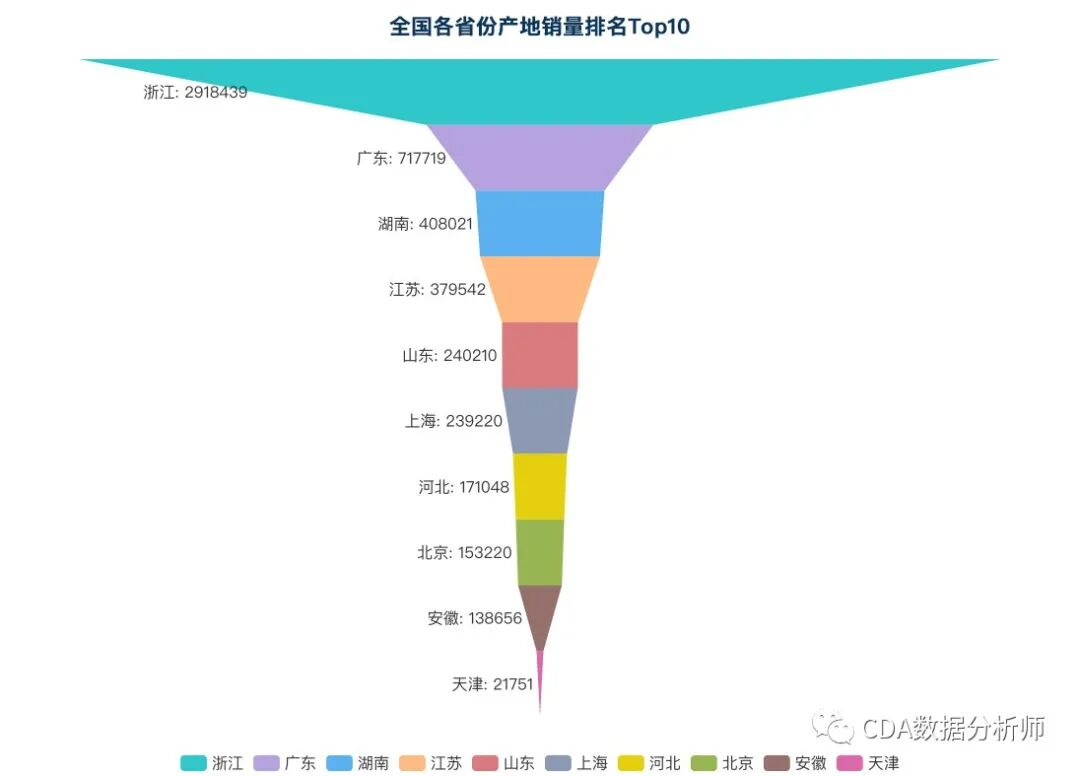`# 计算销量top10 province_top10 = df.groupby('province_name')['purchase_num'].sum().sort_values(ascending=False).head(10)`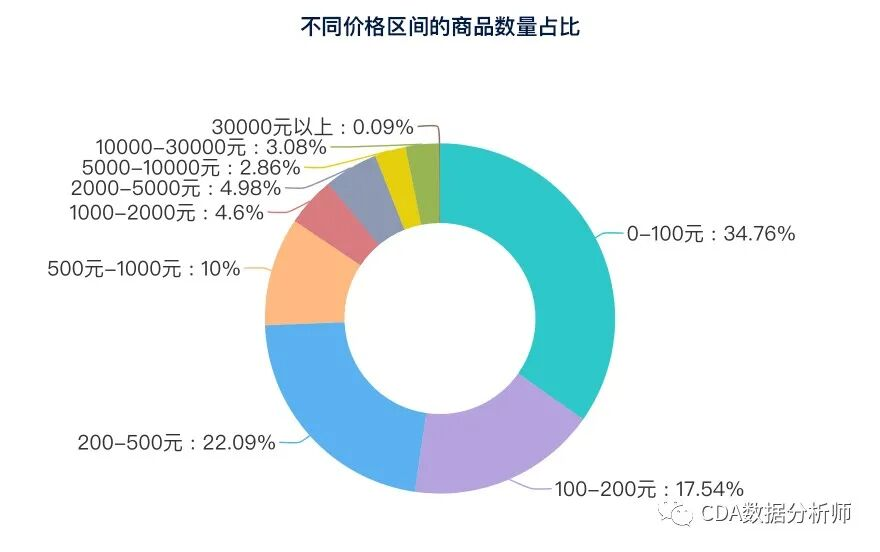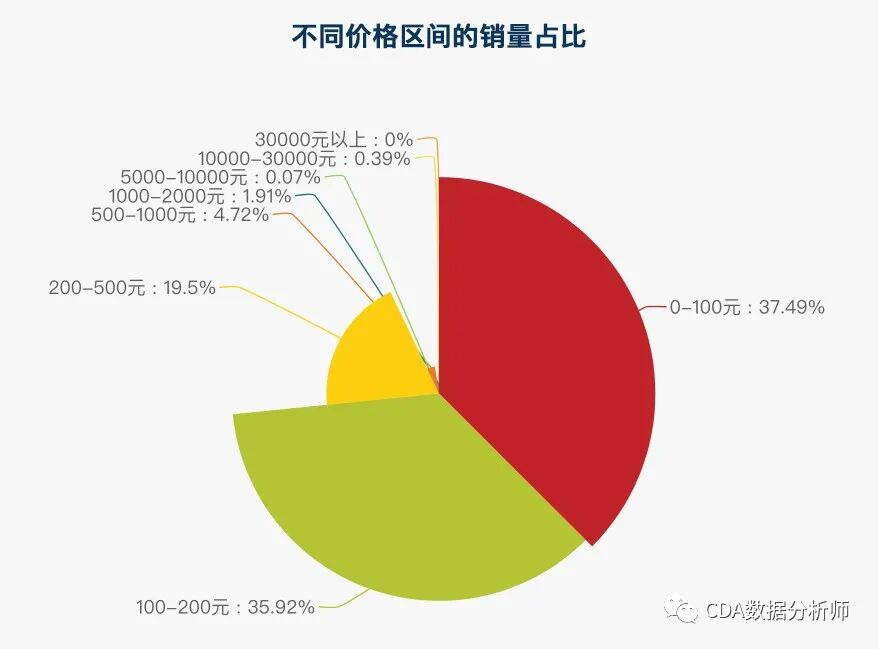OK